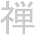## Sunday, January 24, 2010

### Kernel PCA

PCA is a dimension reduction technique for linearly separeted data. In order to deal with data that cannot be linearly separated, one can adopt the kernel trick. This generates the so called Kernel-PCA.

Here you have the code in C++ and Eigen.

1.Works only for a Square matrix as the dataset :(

2.Thanks a lot for the code! Indeed it's a bit buggy, but it's still a very nice starting point. Below is a little fixup that should make it work with any dimensionality.

A quick question: You say "in this toy example we materialize the kernel, which is not necessary". What do you mean exactly? how could we not do that?

ciao
Basilio

void PCA::kernel_pca(MatrixXd & dataPoints, unsigned int dimSpace)
{
int m = dataPoints.rows();
int n = dataPoints.cols();

Kernel k = new Kernel(dataPoints);

// ''centralize''
MatrixXd K_Centralized = k->get()
- MatrixXd::Ones(n, n)*k->get()
- k->get()*MatrixXd::Ones(n, n)
+ MatrixXd::Ones(n, n)*k->get()*MatrixXd::Ones(n, n);
std::cout << "Centralized" << std::endl;

// compute the eigenvalue on the K_Centralized Matrix
EigenSolver m_solve(K_Centralized);
std::cout << "got the eigenvalues, eigenvectors" << std::endl;
VectorXd eigenvalues = m_solve.eigenvalues().real();
MatrixXd eigenVectors = m_solve.eigenvectors().real();

// sort and get the permutation indices
PermutationIndices pi;
for (int i = 0 ; i < n; i++)
pi.push_back(std::make_pair(-eigenvalues(i), i));

std::sort(pi.begin(), pi.end());

// get top eigenvectors
_result = MatrixXd::Zero(n, dimSpace);
for (unsigned int i = 0; i < dimSpace; i++)
{
_result.col(i) = eigenVectors.col(pi[i].second); // permutation indices
}

MatrixXd sqrtE = MatrixXd::Zero(dimSpace, dimSpace);
for (unsigned int i = 0; i < dimSpace; i++)
{
sqrtE(i, i) = sqrt(-pi[i].first);
}

// get the final data projection
_result = (sqrtE * _result.transpose()).transpose();
delete k;
}